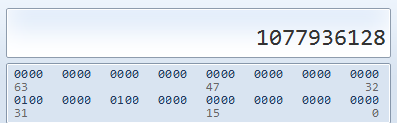# 牛bee的InvSqrt函数-神秘的0x5f3759df

InvSqrt(value)函数相当于1.0/sqrt(value)，只是一个简单地开平方取到数而已，但是下面这个代码竟然比1.0/sqrt(value)快了4倍！

float Q_rsqrt( float number )
{
long i;
float x2, y;
const float threehalfs = 1.5F;

x2 = number * 0.5F;
y   = number;
i   = * ( long * ) &y;   // evil floating point bit level hacking
i   = 0x5f3759df - ( i >> 1 ); // what the fuck?
y   = * ( float * ) &i;
y   = y * ( threehalfs - ( x2 * y * y ) ); // 1st iteration
// y   = y * ( threehalfs - ( x2 * y * y ) ); // 2nd iteration, this can be removed

#ifndef Q3_VM
#ifdef __linux__
assert( !isnan(y) ); // bk010122 - FPE?
#endif
#endif
return y;
}


float InvSqrt(float x)
{
float xhalf = 0.5f*x;
int i = *(int*)&x; // get bits for floating VALUE
i = 0x5f375a86- (i>>1); // gives initial guess y0
x = *(float*)&i; // convert bits BACK to float
x = x*(1.5f-xhalf*x*x); // Newton step, repeating increases accuracy
return x;
}


### 首先第一行：

float xhalf = 0.5f*x;


### 第二行来了：

int i = *(int*)&x; // get bits for floating VALUE


• &x
• 这是取x的地址
• (int*)&x
• 这是把x的地址转成int类型的指针。x本来是float类型的，在内存中存储自然是以float格式存储。但是经过这一操作后，就相当于告诉大家，这里存储的东西不归float管了，归我int管，以后你们要想从这读数据，必须用int格式读。
• 这好比你有一个png图片，你把后缀改成txt，然后双击打开，自然是用记事本打开，而且内容是乱码的。
• *(int*)&x
• 转换后在取出里面的内容，这时是以int类型取出。

3的二进制是11，写成指数是： 1.1 × 2 1 1.1\times2^1

• 符号位是0。（0表示正，1表示负）
• 指数是1，这里因为指数有正有负，所以1+127=128，二进制为10000000。
• 小数位是 . 1 .1 （前面的1省略了，因为它总是1），即 2 − 1 2^{-1} ，为10000000000000000000000（共23位）
• 顺便多解释一下，假如小数位是 . 1001101 .1001101 ，即 2 − 1 + 2 − 4 + 2 − 5 + 2 − 7 + 2^{-1}+2^{-4}+2^{-5}+2^{-7}+ ，则为10011010000000000000000

01000000010000000000000000000000 01000000010000000000000000000000### 更骚的第三行

i = 0x5f375a86- (i>>1); // gives initial guess y0


• i>>1
• i右移一位
• 即二进制为00100000001000000000000000000000
• 十进制为538968064（类似于除2再取整）
• 0x5f375a86
• 神秘的十六进制！
• 十进制：1597463174
• 二进制：1011111001101110101101010000110

。。。写不下去了

09-081万+12-224994
08-109499
11-063473
08-04121
03-03701
10-061万+
12-23789
08-17587
10-223460
11-27263
04-1282
04-0141
03-301993
©️2020 CSDN 皮肤主题: 大白 设计师:CSDN官方博客